# matplotlib

``````export MODULEPATH="\${MODULEPATH}:/hpc/modules/workshop"
cp -R /hpc/examples/workshops/hpc/python-inflammation ~/
cd ~/python-inflammation
srun -p development,htc,mic -c 1 --mem=6G --pty -t 0-2 m2_jupyter_lab
``````

Select the Python 3 install in the Jupyter Lab window.

## Visualizing data

The mathematician Richard Hamming once said, “The purpose of computing is insight, not numbers,” and the best way to develop insight is often to visualize data. Visualization deserves an entire lecture of its own, but we can explore a few features of Python’s `matplotlib` library here. While there is no official plotting library, `matplotlib` is the de facto standard. First, we will import the `pyplot` module from `matplotlib` and use two of its functions to create and display a heat map of our data:

``````import numpy
import matplotlib.pyplot
image = matplotlib.pyplot.imshow(data)
matplotlib.pyplot.show()
``````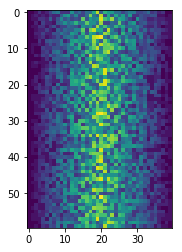Blue pixels in this heat map represent low values, while yellow pixels represent high values. As we can see, inflammation rises and falls over a 40-day period.

## Some IPython Magic

If you’re using a Jupyter notebook, you’ll need to execute the following command in order for your matplotlib images to appear in the notebook when `show()` is called:

``````%matplotlib inline
``````

The `%` indicates an IPython magic function - a function that is only valid within the notebook environment. Note that you only have to execute this function once per notebook.

Let’s take a look at the average inflammation over time:

``````ave_inflammation = numpy.mean(data, axis=0)
ave_plot = matplotlib.pyplot.plot(ave_inflammation)
matplotlib.pyplot.show()
``````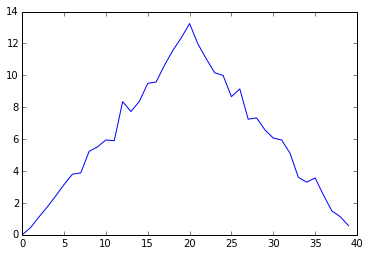Here, we have put the average per day across all patients in the variable `ave_inflammation`, then asked `matplotlib.pyplot` to create and display a line graph of those values. The result is a roughly linear rise and fall, which is suspicious: we might instead expect a sharper rise and slower fall. Let’s have a look at two other statistics:

``````max_plot = matplotlib.pyplot.plot(numpy.max(data, axis=0))
matplotlib.pyplot.show()
``````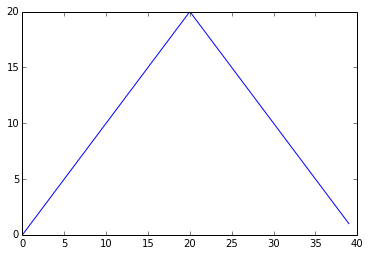``````min_plot = matplotlib.pyplot.plot(numpy.min(data, axis=0))
matplotlib.pyplot.show()
``````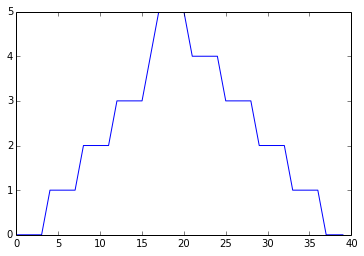The maximum value rises and falls smoothly, while the minimum seems to be a step function. Neither trend seems particularly likely, so either there’s a mistake in our calculations or something is wrong with our data. This insight would have been difficult to reach by examining the numbers themselves without visualization tools.

### Grouping plots

You can group similar plots in a single figure using subplots. This script below uses a number of new commands. The function `matplotlib.pyplot.figure()` creates a space into which we will place all of our plots. The parameter `figsize` tells Python how big to make this space. Each subplot is placed into the figure using its `add_subplot` method. The `add_subplot` method takes 3 parameters. The first denotes how many total rows of subplots there are, the second parameter refers to the total number of subplot columns, and the final parameter denotes which subplot your variable is referencing (left-to-right, top-to-bottom). Each subplot is stored in a different variable (`axes1`, `axes2`, `axes3`). Once a subplot is created, the axes can be titled using the `set_xlabel()` command (or `set_ylabel()`). Here are our three plots side by side:

``````import numpy
import matplotlib.pyplot

fig = matplotlib.pyplot.figure(figsize=(10.0, 3.0))

axes1.set_ylabel('average')
axes1.plot(numpy.mean(data, axis=0))

axes2.set_ylabel('max')
axes2.plot(numpy.max(data, axis=0))

axes3.set_ylabel('min')
axes3.plot(numpy.min(data, axis=0))

fig.tight_layout()

matplotlib.pyplot.show()
``````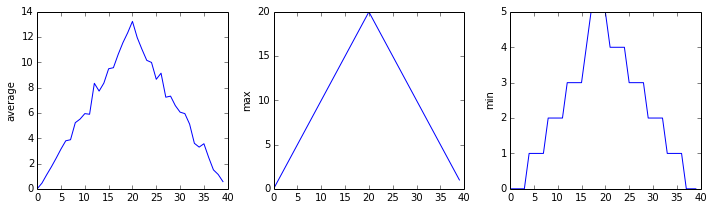The call to `loadtxt` reads our data, and the rest of the program tells the plotting library how large we want the figure to be, that we’re creating three subplots, what to draw for each one, and that we want a tight layout. (If we leave out that call to `fig.tight_layout()`, the graphs will actually be squeezed together more closely.)

What values do the variables `mass` and `age` have after each statement in the following program? Test your answers by executing the commands.

``````mass = 47.5
age = 122
mass = mass * 2.0
age = age - 20
print(mass, age)
``````

## Solution

``````95.0 102
``````

## Sorting Out References

What does the following program print out?

``````first, second = 'Grace', 'Hopper'
third, fourth = second, first
print(third, fourth)
``````

## Solution

``````Hopper Grace
``````

## Slicing Strings

A section of an array is called a slice. We can take slices of character strings as well:

``````element = 'oxygen'
print('first three characters:', element[0:3])
print('last three characters:', element[3:6])
``````
``````first three characters: oxy
last three characters: gen
``````

What is the value of `element[:4]`? What about `element[4:]`? Or `element[:]`?

## Solution

``````oxyg
en
oxygen
``````

What is `element[-1]`? What is `element[-2]`?

## Solution

``````n
e
``````

Given those answers, explain what `element[1:-1]` does.

## Solution

Creates a substring from index 1 up to (not including) the final index, effectively removing the first and last letters from ‘oxygen’

## Thin Slices

The expression `element[3:3]` produces an empty string, i.e., a string that contains no characters. If `data` holds our array of patient data, what does `data[3:3, 4:4]` produce? What about `data[3:3, :]`?

## Solution

``````array([], shape=(0, 0), dtype=float64)
array([], shape=(0, 40), dtype=float64)
``````

## Plot Scaling

Why do all of our plots stop just short of the upper end of our graph?

## Solution

Because matplotlib normally sets x and y axes limits to the min and max of our data (depending on data range)

If we want to change this, we can use the `set_ylim(min, max)` method of each ‘axes’, for example:

``````axes3.set_ylim(0,6)
``````

Update your plotting code to automatically set a more appropriate scale. (Hint: you can make use of the `max` and `min` methods to help.)

## Solution

``````# One method
axes3.set_ylabel('min')
axes3.plot(numpy.min(data, axis=0))
axes3.set_ylim(0,6)
``````

## Solution

``````# A more automated approach
min_data = numpy.min(data, axis=0)
axes3.set_ylabel('min')
axes3.plot(min_data)
axes3.set_ylim(numpy.min(min_data), numpy.max(min_data) * 1.1)
``````

## Drawing Straight Lines

In the center and right subplots above, we expect all lines to look like step functions because non-integer value are not realistic for the minimum and maximum values. However, you can see that the lines are not always vertical or horizontal, and in particular the step function in the subplot on the right looks slanted. Why is this?

## Solution

Because matplotlib interpolates (draws a straight line) between the points. One way to do avoid this is to use the Matplotlib `drawstyle` option:

``````import numpy
import matplotlib.pyplot

fig = matplotlib.pyplot.figure(figsize=(10.0, 3.0))

axes1.set_ylabel('average')
axes1.plot(numpy.mean(data, axis=0), drawstyle='steps-mid')

axes2.set_ylabel('max')
axes2.plot(numpy.max(data, axis=0), drawstyle='steps-mid')

axes3.set_ylabel('min')
axes3.plot(numpy.min(data, axis=0), drawstyle='steps-mid')

fig.tight_layout()

matplotlib.pyplot.show()
``````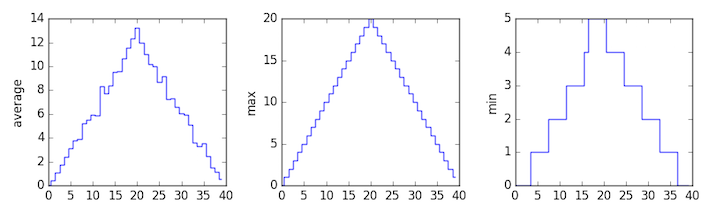Create a plot showing the standard deviation (`numpy.std`) of the inflammation data for each day across all patients.

## Solution

``````std_plot = matplotlib.pyplot.plot(numpy.std(data, axis=0))
matplotlib.pyplot.show()
``````

## Moving Plots Around

Modify the program to display the three plots on top of one another instead of side by side.

## Solution

``````import numpy
import matplotlib.pyplot

# change figsize (swap width and height)
fig = matplotlib.pyplot.figure(figsize=(3.0, 10.0))

# change add_subplot (swap first two parameters)

axes1.set_ylabel('average')
axes1.plot(numpy.mean(data, axis=0))

axes2.set_ylabel('max')
axes2.plot(numpy.max(data, axis=0))

axes3.set_ylabel('min')
axes3.plot(numpy.min(data, axis=0))

fig.tight_layout()

matplotlib.pyplot.show()
``````

## Stacking Arrays

Arrays can be concatenated and stacked on top of one another, using NumPy’s `vstack` and `hstack` functions for vertical and horizontal stacking, respectively.

``````import numpy

A = numpy.array([[1,2,3], [4,5,6], [7, 8, 9]])
print('A = ')
print(A)

B = numpy.hstack([A, A])
print('B = ')
print(B)

C = numpy.vstack([A, A])
print('C = ')
print(C)
``````
``````A =
[[1 2 3]
[4 5 6]
[7 8 9]]
B =
[[1 2 3 1 2 3]
[4 5 6 4 5 6]
[7 8 9 7 8 9]]
C =
[[1 2 3]
[4 5 6]
[7 8 9]
[1 2 3]
[4 5 6]
[7 8 9]]
``````

Write some additional code that slices the first and last columns of `A`, and stacks them into a 3x2 array. Make sure to `print` the results to verify your solution.

## Solution

A ‘gotcha’ with array indexing is that singleton dimensions are dropped by default. That means `A[:, 0]` is a one dimensional array, which won’t stack as desired. To preserve singleton dimensions, the index itself can be a slice or array. For example, `A[:, :1]` returns a two dimensional array with one singleton dimension (i.e. a column vector).

``````D = numpy.hstack((A[:, :1], A[:, -1:]))
print('D = ')
print(D)
``````
``````D =
[[1 3]
[4 6]
[7 9]]
``````

## Solution

An alternative way to achieve the same result is to use Numpy’s delete function to remove the second column of A.

``````D = numpy.delete(A, 1, 1)
print('D = ')
print(D)
``````
``````D =
[[1 3]
[4 6]
[7 9]]
``````

## Change In Inflammation

This patient data is longitudinal in the sense that each row represents a series of observations relating to one individual. This means that the change in inflammation over time is a meaningful concept.

The `numpy.diff()` function takes a NumPy array and returns the differences between two successive values along a specified axis. For example, a NumPy array that looks like this:

``````npdiff = numpy.array([ 0,  2,  5,  9, 14])
``````

Calling `numpy.diff(npdiff)` would do the following calculations and put the answers in another array.

``````[ 2 - 0, 5 - 2, 9 - 5, 14 - 9 ]
``````
``````numpy.diff(npdiff)
``````
``````array([2, 3, 4, 5])
``````

Which axis would it make sense to use this function along?

## Solution

Since the row axis (0) is patients, it does not make sense to get the difference between two arbitrary patients. The column axis (1) is in days, so the difference is the change in inflammation – a meaningful concept.

``````numpy.diff(data, axis=1)
``````

If the shape of an individual data file is `(60, 40)` (60 rows and 40 columns), what would the shape of the array be after you run the `diff()` function and why?

## Solution

The shape will be `(60, 39)` because there is one fewer difference between columns than there are columns in the data.

How would you find the largest change in inflammation for each patient? Does it matter if the change in inflammation is an increase or a decrease?

## Solution

By using the `numpy.max()` function after you apply the `numpy.diff()` function, you will get the largest difference between days.

``````numpy.max(numpy.diff(data, axis=1), axis=1)
``````
``````array([  7.,  12.,  11.,  10.,  11.,  13.,  10.,   8.,  10.,  10.,   7.,
7.,  13.,   7.,  10.,  10.,   8.,  10.,   9.,  10.,  13.,   7.,
12.,   9.,  12.,  11.,  10.,  10.,   7.,  10.,  11.,  10.,   8.,
11.,  12.,  10.,   9.,  10.,  13.,  10.,   7.,   7.,  10.,  13.,
12.,   8.,   8.,  10.,  10.,   9.,   8.,  13.,  10.,   7.,  10.,
8.,  12.,  10.,   7.,  12.])
``````

If inflammation values decrease along an axis, then the difference from one element to the next will be negative. If you are interested in the magnitude of the change and not the direction, the `numpy.absolute()` function will provide that.

Notice the difference if you get the largest absolute difference between readings.

``````numpy.max(numpy.absolute(numpy.diff(data, axis=1)), axis=1)
``````
``````array([ 12.,  14.,  11.,  13.,  11.,  13.,  10.,  12.,  10.,  10.,  10.,
12.,  13.,  10.,  11.,  10.,  12.,  13.,   9.,  10.,  13.,   9.,
12.,   9.,  12.,  11.,  10.,  13.,   9.,  13.,  11.,  11.,   8.,
11.,  12.,  13.,   9.,  10.,  13.,  11.,  11.,  13.,  11.,  13.,
13.,  10.,   9.,  10.,  10.,   9.,   9.,  13.,  10.,   9.,  10.,
11.,  13.,  10.,  10.,  12.])
``````

Based on the tutorials by the The Carpentries.